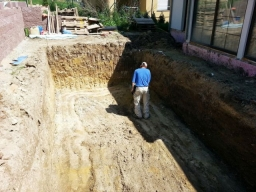# Cross-sectional 18973

Calculate the cross-sectional area of the trapezoidal channel irrigation channel if its upper width is 7.9 m, its bottom width is 5.5 m, and its depth is 2.8 m.

S =  18.76 m2

### Step-by-step explanation:Did you find an error or inaccuracy? Feel free to write us. Thank you!

Tips for related online calculators
Need help calculating sum, simplifying, or multiplying fractions? Try our fraction calculator.

#### Grade of the word problem:

We encourage you to watch this tutorial video on this math problem: